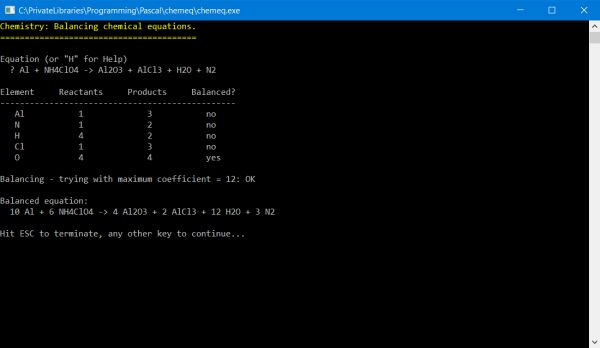# Computing: Free Pascal Programming

## Balancing chemical equations.

Description: The program calculates the coefficients of the reactants and products of a chemical reaction in order to balance the equation entered by the user. Enter the (unbalanced) equation using the format:
reactant1 + reactant2 ... -> product1 + product 2 ...
Entering "H" instead, displays a short help text.

Programming notes: The program uses a brute force approach to determine the coefficients. This may result in thousands and thousands of calculations to be done. Whereas for "normal" equations, this doesn't really matter and the answer is more or less instantaneous, for complicated equations, containing 6 molecules and coefficients greater than 10, it may take time. Al + NH4ClO4 -> Al2O3 + AlCl3 + H2O + N2 (balanced: 10Al + 6NH4ClO4 -> 4Al2O3 + 2AlCl3 + 12H2O + 3N2) took some 15 secs on my machine; but these "extreme cases" are very rare in practice.

Coefficients are calculated by generating all size (reactants + products) combinations (including repetitions) of the integers from 1 to a presupposed maximum coefficient value. The program first tries with CoeffMax = 6, then CoeffMax = 12 and finally, if the equation is still not balanced, asks the user if it should continue trying. This has been done to limit calculation time on slower computers, considering that it's rather rare to have equations with coefficient maxima greater than 6.

Version 1.1 (August 2018): Bug fixed: Equations with molecules containing "{Atom}10" or "({Atoms})10" are correctly balanced now.

Improvements: Gaining lots of speed by eliminating those combinations which can't be the searched coefficients (e.g. if 1 1 1 1 is not the solution, 2 2 2 2 and 3 3 3 3 can't be either). Another way to limit the number of calculations would be to sort the combinations differently (2 2 3 1 is lots more probable than 1 1 1 12...)

Issue: When I used the program one of these days (February 2022), I noticed that there is something wrong: Not arrived to balance simple equations like H2SO4 + NaOH -> NaSO4 + H2O or HCl + Ca(OH)2 -> CaCl + H2O. So, it seems that the program is not working at all. Bizarre, if you consider the screenshot below!? Anyway, I've removed the program from the download directories...

Free Pascal features: Recursion, string extraction, complex Pascal data structures.

Screenshot: